Here we are providing Class 11 chemistry Important Extra Questions and Answers Chapter 6 Thermodynamics. Chemistry Class 11 Important Questions are the best resource for students which helps in Class 11 board exams.

## Class 11 Chemistry Chapter 6 Important Extra Questions Thermodynamics

### Thermodynamics Important Extra Questions Very Short Answer Type

Question 1.
Under what conditions the heat evolved or absorbed is equal to the internal energy change?
At constant volume.

Question 2.
What is the sign of AH for endothermic reactions and why?
AH is positive as ΔH = Hp – Hr and Hr < Hp.

Question 3.
What is the relationship between the standard enthalpy of formation and the enthalpy of a compound?
They are equal.

Question 4.
Why enthalpy of neutralization of HF is greater than 57.1 kJ mol-1?
This is due to the high hydration energy of fluoride ions.

Question 5.
What are the specific heat capacity and molar heat capacity for water?
Specific heat capacity for H2O = 4.18 JK-1 g-1
Molar heat capacity for H2O = 4.18 × 18 = 75.24 JK-1 mol-1.

Question 6.
Why enthalpy of neutralization is less if either the acid or the base or both are weak?
A part of the heat is used up for dissociation of the weak acid or weak base or both.

Question 7.
What do you mean by a system?
A specified part of the universe that is under thermodynamic observation is called a system.

Question 8.
Define a cyclic process.
A process in which a system undergoes a series of changes and ultimately returns to the original state is called a cyclic process. For a cyclic process; ΔU = 0.

Question 9.
Why the entropy of a diamond is less than that of graphite?
Diamond is more compact than graphite.

Question 10.
Under what conditions, ΔH of a process is equal to ΔU?
At constant temperature and constant volume.

Question 11.
Is the enthalpy of neutralization of HCl is same as that of H2S04? If so, why?
Yes. Because both are strong acids and ionized almost completely in aqueous solutions.

Question 12.
Which is a better fuel for the animal body: proteins or carbohydrates?
Carbohydrates. They have high calorific value.

Question 13.
Is the experimental determination of enthalpy of formation of CH4 possible?
No.

An online enthalpy calculator is specially designed to calculate exact amount of enthalpy generated in a thermodynamic system.

Question 14.
Can we calculate ΔH of every process from the bond energy data of reactants and products?
No.

Question 15.
Define enthalpy of fusion.
It is defined as the heat change when one mole of solid changes to its liquid form at its melting point. ‘

Question 16.
For the reaction NaCl (aq) + AgNO3 (aq) → AgCl(s) + NaNO3(aq), will ΔH be greater than, equal to or less than ΔE?
ΔH will be ΔE.

Question 17.
Write the mathematical relationship between heat, internal energy, and work done on the system.
ΔE = q + w.

Question 18.
What is the limitation of the I law of thermodynamics?
It cannot tell us about the direction of the process

Question 19.
What is the relationship between ΔH and ΔE?
ΔH = ΔE + PΔV = ΔE + ΔngRT.

Question 20.
State the I law of thermodynamics.
OR
State the Law of conservation of energy.
The energy of an isolated system remains conserved.
OR
The energy can neither be created nor destroyed, though it can be converted from one form into another.

Question 21.
Which of the following is a state function?
(i) height of a hill
(ii) distance traveled in climbing the hill
(iii) energy consumed in climbing the hill.
Energy consumed in climbing the hill.

Question 22.
What is the internal energy of one mole of a noble gas?
U = $$\frac{3}{2}$$RT.

Question 23.
Which of the following are state functions?
(i) q
(ii) heat capacity
(iii) specific heat capacity
(iv) ΔH and ΔU.
(iii) specific heat capacity and
(iv) ΔH and ΔU

Question 24.
Name the two most common modes by which a system and surroundings exchange their energy?
Heat and work.

Question 25.
What will happen to internal energy if work is done by the system?
The internal energy of the system will decrease.

Question 26.
How many times is the molar heat capacity greater than the specific heat capacity of water?
18 times.

Question 27.
Neither q nor w is a state function but q + w is a state function. Why?
q + w is equal to ΔU, which is a state function.

Question 28.
Name the state function Which remains constant during an isothermal change.
Temperature.

Question 29.
What is the value of enthalpy of neutralization of a strong acid and a strong base?
– 57.1 kJ/gm equivalent.

Question 30.
Out of 1 mole of H2O(g) and 1 mole of H2O(l) which one will have greater entropy?
H2O(g) will have greater entropy.

Question 31.
When a substance is said to be in its standard state?
When it is present at 298 K and under one atmospheric pressure.

Question 32.
What is the entropy of the formation of an element in its standard state?
By convention, the enthalpies (heats) of the formation of all elements in their most stable form (standard state) is taken as zero.

Question 33.
What is the physical significance of free energy change of a system?
The decrease in free energy (- ΔG) is a measure of useful work or network obtainable during the process at constant temp and pressure.

Question 34.
Why is it essential to mention the physical state of reactants and products in thermochemical reactions?
It is because the physical state of reactants and products also contributes significantly to the value ΔU or ΔH.

Question 35.
Name two intensive and extensive properties of a system.

• Intensive properties: Viscosity, refractive index.
• Extensive properties: Mass, volume, heat capacity, etc.

Question 36.
What is fuel value or calorific value?
It is defined as the amount of heat released when 1 gm of fuel or food is burnt completely in air or oxygen.

Question 37.
Is the process of diffusion of gases enthalpy driven or entropy-driven?
It is an entropy-driven process.

Question 38.
The value of ΔHsol of NaNO3 is positive, yet the dissolution is spontaneous, why?
There is a large increase in entropy i.e., it is an entropy-driven process.

Question 39.
Write a thermochemical equation of enthalpy of combustion of methanol.
2CH3OH(l) + 3O2(g) → 2CO2(g) + 4H2O(1); ΔH = – Q kJ
where Q kJ mol-1 is the enthalpy of combustion.

Question 40.
What is the enthalpy of the formation of Cl2?
Enthalpy of formation of a homonuclear molecule like Cl2 is zero.

Question 41.
For a reaction also ΔH and ΔS are positive. What is the condition that this reaction occurs spontaneously?
To make ΔG negative TΔS > ΔH.

Question 42.
Arrange the following fuels in order of increasing fuel efficiency; kerosene, diesel oil, wax, natural gas.
Lower hydrocarbons have higher calorific value and thus are more efficient.
wax < diesel oil < kerosene < natural gas.

Question 43.
What is the sign of ΔS when N2 and H2 combine to form NH3?
ΔS is negative as the number of molecules decreases.

Question 44.
How will compare the efficiency of the three given fuels?
By comparing their calorific values. Larger the calorific value, the greater the fuel efficiency.

Question 45.
How is a non-spontaneous process made spontaneous?
By continuously supplying energy to it from outside.

Question 46.
What is the expression for entropy change for a phase transition? ‘
ΔS = $$\frac{q_{\mathrm{rev}}}{\mathrm{T}}$$

Question 47.
Give an example of an isolated system.
Thermos.

Question 48.
Is the enthalpy of formation of SnCl2(s) the same as that of ZnCl2(s)?
No. They are different.

Question 49.
Why we usually study enthalpy change and not internal energy change?
Most of the processes including reactions are carried out in open vessels at constant pressure.

Question 50.
What is the enthalpy of one mole of a noble gas?
H = U + PV = $$\frac{3}{2}$$ RT + RT = $$\frac{5}{2}$$ RT.

Question 51.
Which of the thermodynamic properties out of U, S, T, P, V, H, and G are intensive properties and why?
T and P, because they depend only upon the nature of the substance.

Question 52.
What is the relationship between qp and qv?
qp = qv + Δng RT, where Δn = np– nr (gaseous).

Question 53.
What is the Gibb’s Helmholtz equation?
ΔG = ΔH – TΔS, where ΔG, ΔH, and ΔS are free energy change, enthalpy change, and entropy change respectively.

Question 54.
Comment on the bond energies of four C-H bonds present in CH4?
The bond energies of 1st, 2nd, 3rd, and 4th C-H bonds are not equal and so average values are taken.

Question 55.
What is the main limitation of the first law of thermodynamics?
It cannot predict the spontaneity of a process.

Question 56.
What is entropy?
Entropy is a measure of the randomness/disorder of a system.

Question 57.
A reversible reaction has ΔG° negative for forwarding reaction. What will be the sign of ΔG° for the backward reaction?
Negative.

Question 58.
What is the effect of increasing temperature on the entropy of a substance?
It increases.

Question 59.
When is the entropy of a perfectly crystalline solid zero?
At absolute zero (O.K).

Question 60.
In which no eat flow between the system and surroundings.

### Thermodynamics Important Extra Questions Short Answer Type

Question 1.
Ice is lighter than water, but the entropy of ice is less than that of water. Explain.
Water is the liquid form while ice is its solid form. Molecular motion in ice is restricted than in water, i.e., a disorder in ice is restricted than water, i.e., a disorder in ice is less than in water.

Question 2.
Define spontaneity or-feasibility of a process.
Spontaneity or feasibility of a process means its inherent tendency to occur on its own in a particular direction under a given set of conditions.

Question 3.
Enthalpy of neutralization of CH3COOH and NaOH is 55.9 kJ. What is the value of ΔH for ionization of CH3COOH?
The heat of neutralization of strong acid and strong base + ΔH of ionization of CH3COOH = Enthalpy of neutralization of CH3COOH and NaOH
∴ – 57.1 kJ + ΔH of ionisation of CH3COOH = – 55.9 kJ
∴ ΔH of ionisation of CH3COOH = (- 55.9 + 57.1) kJ
= 1.2 kJ.

Question 4.
When 1 gm of liquid naphthalene (C10H8) solidifies, 150 J of heat is evolved. What is the enthalpy of fusion of C10H8?
ΔHsolidifcation = – 150 × 128 = – 19200 J = – 19.2 kJ
[∵ M.wt.of C10H8 = 128]

Question 5.
Why most of the exothermic processes (reactions) are spontaneous?
ΔG = ΔH – TΔS; For exothermic reactions,
ΔH is -ve For a spontaneous process ΔG is to be -ve.

Thus decrease in enthalpy (- AH) contributes significantly to the driving force (To make ΔG negative).

Question 6.
What is meant by the term state function? Give examples.
A state function is a thermodynamic property that depends upon the state of the system and is independent of the path followed to bring about the change. Internal energy change (ΔU), enthalpy change (ΔH) entropy change (ΔS), and free energy change (ΔG) are examples.

Question 7.
The enthalpy of combustion of sulfur is 297 kJ.
Write the thermochemical equation for the combustion of sulfur. What is the value of ΔfH of SO2?
S(s) + O2(g) → SO2(g); ΔfH = – 297 kJ
∴ ΔH of SO2 = – 297 kJ mol-1

Question 8.
What would be the heat released when 0.35 mol of HC1 in solution is neutralized by 0.25 mol of NaOH solution?
HCl and NaOH being strong acid and strong- base is completely ionization in dilute aqueous solutions. The net reaction is H + (0.25 mol) + OH (0.25. mol) → H2O (0.25 mol)

Now the heat of neutralization of 1 mole of a strong acid is – 57.1 kJ
∴ The heat released will be 57.1 × 0.25 kJ = 14.27 kJ.

Question 9.
Predict which of the following entropy increases/ decreases?
(i) A liquid crystallizes into a solid.
After crystallization molecules attain an ordered state and therefore entropy decreases.

(ii) Temperature bf a crystalline solid is raised from 0 K to 115 K.
When the temperature is raised, a disorder in molecules increases, and therefore entropy increases.

(iii) 2NaHCO3(s) → Na2CO3(s) + CO2(g) + H2O(g)
The reactant is solid and hence has low entropy. Among the products there are two gases and be solid, therefore products represent a condition of higher entropy.

(iv) H2(g) → 2H(g).
Here 2 moles of H atoms have higher entropy than one mole of the hydrogen molecule.

Question 10.
Discuss the effect of temperature on the spontaneity of reactions.
Effect of temperature on the spontaneity of reactions: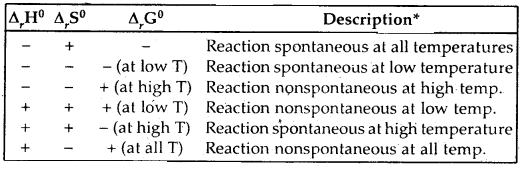The terms low temperature and high temperature are relative. For a particular reaction, the high temperature could even mean room temperature.

Question 11.
What is the most important condition for a process to be reversible in thermodynamics?
The process should be carried out infinitesimally slowly or the driving force should be infinitesimally greater than the opposing force.

Question 12.
Why heat is not a state function?
According to first law of Thermodynamics; ΔU = q + w or q – = ΔU – w. As ΔU is a state function, but w is not a state function, therefore q is also not a state function.

Question 13.
Why the absolute value of enthalpy cannot be determined?
As H = U + PV
The absolute value of U – the internal energy cannot be determined as it depends upon various factors whose value, cannot be determined.
∴ The absolute value of H cannot be determined.

Question 14.
Which of the following is/are exothermic and which are endothermic?
(i) Ca(g) → Ca2+(g) + 2e
Endothermic (Ionisation enthalpy is required)

(ii) O(g) + e → O(g) .
Exothermic (first electron affinity-energy is released)

(iii) N2-(g) + e- → N3-(g).
Endothermic (higher electron affinities are required).

Question 15.
Calculate Δr Gθ for the conversion of oxygen to ozone, $$\frac{3}{2}$$O2(g) → O3(g) at 298 K, if Kp for this conversion is 2.47 × 10-29
Δr Gθ = – 2.303RT log Kp
and R = 8.314 JK-1 mol-1
∴ Δr Gθ = – 2.303 × 8.314 × 298 log 2.47 × 10-29
= 163000 j mol-1 = 163 kJ mol-1.

Question 16.
Find the value of the equilibrium constant for the following conversion reaction at 298 K.Δr Gθ = – 13.6 kJ mol-1.
r Gθ = 2.303 RT log K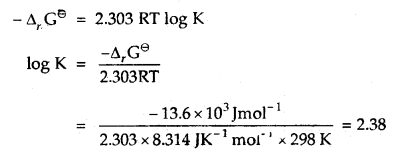Question 17.
At 60°C, N2O4 is 50% dissociated. Calculate Δr Gθ this temperature and at one atmospheric pressure.
N2O4(g) ⇌ 2NO2(g)
If N2O4 is 50% dissociated, the mole fraction of both the substances are given byQuestion 18.
Calculate the heat released when 0.5 moles of the nitric acid solution is mixed with 0.2 moles of potassium hydroxide solution.
HNO3 is a strong acid and KOH is a strong base. Therefore, both are completely ionized in water. The net reaction is∴ Heat evolved = – 57.1 × 0.2 = – 11.42 kJ.

Question 19.
Define Hess’s Law of Constant Heat Summation.
The enthalpy change for a reaction remains the same whether it proceeds in one step or in series of steps all’ measurements being done under similar conditions of temperature.

This law is a corollary from I Law of Thermodynamics.

Question 20.
What are the applications of Hess’s Law of constant heat summation?

1. It helps to calculate the enthalpies of formation of those compounds which Cannot be determined experimentally.
2. It helps to determine the enthalpy of allotropic transformations like C(graphite) → C (diamond).
3. It helps to calculate the enthalpy of hydration.

Question 21.
Calculate the maximum work obtained when 0.75 mol of an ideal gas expands isothermally and reversible at 27°C from a volume of 15 L to 25 L.
For an isothermal reversible expansion of an ideal gas
w = – nRT log $$\frac{\mathrm{V}_{2}}{\mathrm{~V}_{1}}$$ = – 2.303 nRT log $$\frac{\mathrm{V}_{2}}{\mathrm{~V}_{1}}$$

Putting n = 0.75 mol; V1 = 15 L; V2 = 25 L, T = 27 + 273 = 300 K R = 8.314 JK-1 mol-1.
w = – 2.303 × 0.75 × 8.314 × 300 log $$\frac{25}{15}$$
= – 955.5 J.

Question 22.
What are heat capacities at constant volume and constant pressure? What is the relationship between them?
Heat capacity at constant volume (Cv): Heat supplied to a system to raise its temperature through 1°C keeping the volume of the system constant is called its heat capacity at constant volume (Cv).

Heat capacity at constant pressure (Cp): Heat supplied to a system to raise its temperature through 1°C keeping the external pressure constant is called its heat capacity at constant pressure (Cp).

Relationship between Cp and Cv: Cp – Cv = R.

Question 23.
Explain what do you mean by a reversible process.
A process or a change is said to be reversible if it can be reversed at any moment by an infinitesimal change. It proceeds infinitesimally slowly by a series of equilibrium states such that the system and surroundings are always in near equilibrium with each other.

Question 24.
Two liters of an ideal gas at a pressure of 10 atm expands isothermally into a vacuum until its. total volume is 10 liters. How much heat is absorbed and how much work is done in the expansion?
We have q = – w: pext(10 – 2) = 0 × 8 = 0
No. work is done; No heat is absorbed.

Question 25.
Define (i) Molar enthalpy of fusion
The enthalpy change that accompanies the melting of one mole of a solid substance at its melting point is called the articular enthalpy of fusion.

(ii) Molar enthalpy of vaporisation.
The amount of heat required to vaporize one mole of a liquid at constant temperature and under standard pressure (1 bar) is called molar enthalpy of vaporization.

Question 26.
How will you arrive at the relationship qp = qv + ΔngRT?
Enthalpy change ΔH = qp; where qp = heat change at constant pressure,
Internal energy change ΔU = qv; where qv = heat change at. constant volume.

Now ΔH = ΔU + PΔV
For ideal gases PV = nRT
∴ ΔH = ΔU + (PV2 – PV1)
= ΔU + P(V2 – V1) = ΔU + (n2RT – n1RT)
= ΔU + RT(n2 – n1) = ΔU + ΔngRT
or
qp = qv + ΔngRT

Question 27.
Define
(i) Specific heat capacity
Specific heat capacity: It is the amount of heat required to raise the temperature of 1 gram of the substance through 1°C.

(ii) molar heat capacity.
Molar heat capacity: It is the amount of heat required to raise the temperature of one mole of the substance through 1 °C.

Question 28.
Derive the relationship Cp – Cv = R.Question 28.
Derive the expression -ΔG = w non-expansion
From I law of thermodynamics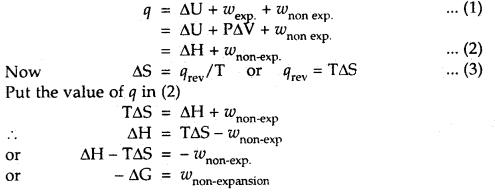Question 29.
How does the sign of G help in predicting the spontaneity/non-spontaneity of a process?

1. IF ΔG is negative, the process is spontaneous.
2. If ΔG = O, the process does not occur and the system is in equilibrium.
3. If ΔG is positive, the process does not proceed in the forward direction.

Question 30.
An exothermic reaction A B is spontaneous in the backward direction. What will be the sign of ΔS for the forward direction?
The backward reaction will be endothermic. Thus, the energy factor opposes the backward reaction., As the backward reaction is spontaneous, the randomness factor must favor i.e., ΔS will be positive for the backward reaction or it will be negative for the forward direction.

### Thermodynamics Important Extra Questions Long Answer Type

Question 1.
Define
(i) Standard enthalpy of formation.
Standard enthalpy of formation: The heat change accompanying the formation of 1 mole df a substance from its elements in their most stable state of aggregation is called its standard enthalpy of formation.
H2(g) + $$\frac{1}{2}$$O2(g) H2O(l); Δf He = 285.8 kJ mol-1

(ii) Standard enthalpy of combustion
Standard enthalpy of combustion: It is the heat change accompanying the complete combustion or burning of one mole of a substance in its standard state in excess of air or oxygen.
C4H10(g) + $$\frac{13}{2}$$O2(g) → 4CO2(g) + 5H2O(1); ΔHθ = – 2658.0 kJ mol-1.

(iii) Enthalpy of atomization
Enthalpy of atomization: It is defined as the enthalpy change accompanying the breaking of one mole of a substance completely into its atoms in the gas phase.
H2(g) → 2H(g) ΔcHe = 435.0 kJ mol-1

(iv) Enthalpy of solution
Enthalpy of solution: It is defined as the heat change when one mole of a substance dissolves in a specified amount of the solvent. The enthalpy of solution at infinite dilution is the enthalpy change observed on dissolving 2 moles of the substance in an infinite amount of the solvent.

(v) Lattice enthalpy
Lattice Enthalpy: The lattice enthalpy of an ionic compound is the enthalpy change that occurs when one mole of an ionic compound dissociates into its ions in a gaseous state.(vi) Thermochemical equation.
Thermochemical Equation: A balanced chemical equation together with the value of its A^H is called a thermochemical equation.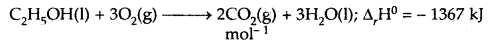The above equation describes the combustion of liquid ethanol. The negative sign indicates that tills are an exothermic reaction. We specify the physical state along with the allotropic state of the substance in a thermochemical equation.

### Thermodynamics Important Extra Questions Numerical Problems

Question 1.
For the equilibrium PCl5(g) ⇌ PCl3(g) + Cl2(g) at 298 K, Kc = 1.8 × 10-7. What is ΔG° for the reaction? (R = 8.314 JK-1 mol-1).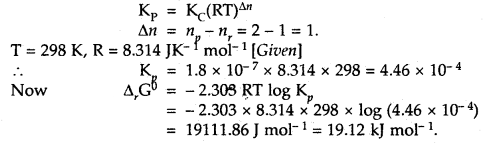Question 2.
Calculate the equilibrium constant, K, for the following reaction at 400 K?
2NOCl(g) ⇌ 2NO(g) + Cl2(g)
Given that ΔrH° = 80.0 kJ mol-1 and ΔrS° = 120 JK-1 mol-1.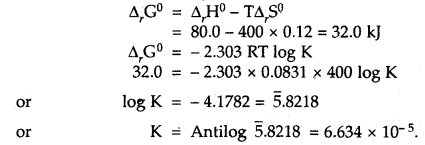Question 3.
Calculate the standard entropy change for the reaction X ⇌ Y if the value of ΔH° = 28.40 kJ and equilibrium constant is 1.8 × 10-7 at 298 K and ΔrG° = 38.484 kJ.= -33.8 JK-1 mol-1

Question 4.
Calculate the enthalpy of formation of methane, given that the enthalpies of combustion of methane, graphite, and hydrogen are 890.2 kJ, 393.4 kJ, and 285.7 kJ mol-1 respectively.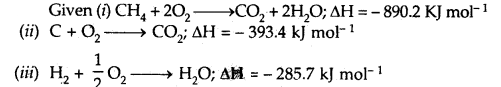Multiply equation (iii) by 2, add it to equation (ii) and subtract equation (i) from their sum
C + 2H2 → CH4 .
ΔH = – 393.4 + 2(-285.7) – (-890.2)
= – 74.6 kJ mol-1
Hence the heat of formation of methane (CH4) is
ΔfH = – 74.6 kJ mol-1.

Question 5.
CO is allowed to expand isothermally and reversibly from 10 m3 to 20 m3 at 300 K and work obtained is 4.75 KJ. Calculate the number of moles of carbon monoxide (CO). R = 8.314 JK-1 mol-1.Question 6.
Two moles of an ideal gas initially at 27°C and one atm pressure are compressed isothermally and reversible till the final pressure of the gas is 10 atm. Calculate q, w, and AU for the process.
Here n = 2; p1 = 1 atm; P2 = 10 atm; T = 300 K
w = 2.303 nRT log $$\frac{\mathrm{P}_{2}}{\mathrm{P}_{1}}$$
= 2.303 × 2 × 8.314 JK mol-1 × 300 K × log $$\frac{10}{1}$$
= 11488 J .

For isothermal compression of ideal gas
ΔU = 0
Further, ΔU = q + w
∴ q = -w = 11488 J.

Question 7.
The heat of combustion of benzene in a bomb calorimeter (i.e. at constant volume) was found to be 3263.9 kJ mol-1 at 25°C. Calculate the heat of combustion of benzene at constant pressure.
The given reaction is
C6H6(l) + $$\frac{15}{2}$$ O2(g) → 6CO2(g) + 3H2O(l)
C6H6 is a liquid and H20 is a liquid at 25°C.Question 8.
When 0.532 g of benzene (C6H6) with boiling point 353 K is burnt with an excess of O2 in a calorimeter, 22.3 kJ of heat is given out. Calculate ΔH for the combustion process (R = 8.31 JK-1 mol-1)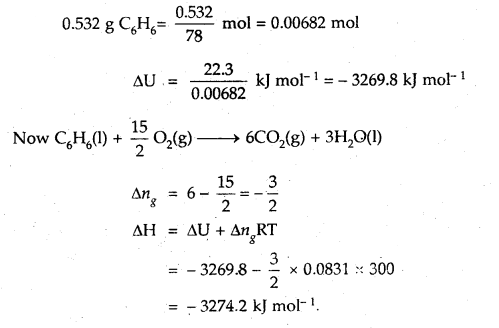Question 9.
Specific heat of an elementary gas is found to be 0.313 Jat constant volume. If the molar mass of the gas is 40 g mol-1, what is the atomicity of the gas? R = 8.31 JK-1 mol-1.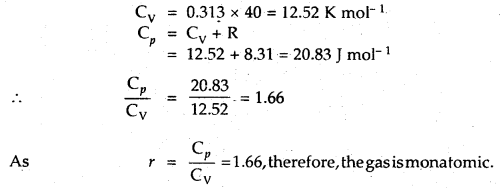Question 10.
Calculate the energy needed to raise the temperature of 10.0 g of iron from 25° C to 500°C if the specific heat of iron is 0.45 J (0°C)-1g-1.
Energy needed (q) = m × C × ΔT
= 10.0 × 0.45 × (500 – 25) J
= 2137.5 J

Question 11.
Calculate the enthalpy of formation of carbon disulfide given that the enthalpy of combustion of it is 110.2 kJ mol-1 and those of sulfur and carbon are 297.4 kJ and 394.5 kJ/g atoms respectively.
Our aim is C(s) + 2S(s) → CS2(l); ΔH =?
Given (i) CS2(l) + 3O2(g) → CO2(g) + 2SO2(g); ΔH = – 110.2 kJ mol-1
(ii) S(s) + O2(g) → SO2(g); ΔH = – 297.4 kj mol-1
(iii) C(s) + O2(g) → CO2(g); ΔH= – 394.5 kj mol-1

Add (iii) + 2(ii) and subtract (i), it gives, on rearranging
C(s) + 2S(s) → CS2(l);
ΔH = (- 394.5) +- 2(- 297.4) – (- 110.2)
= -879.1 kj mol-1

Thus the enthalpy of formation of CS2 = – 879.1 kJ mol-1

Question 12.
There are two crystalline forms of PbO; one is yellow and the other is red. The standard enthalpies of formation of these two forms are – 217.3 and – 219.0 kJ mol-1 respectively. Calculate the enthalpy change for the solid-solid phase transition:
PbO (yellow) → PbO(red)
Our aim is PbO (yellow) → PbO (rpd); ΔH =?
Given (i) PbO(s) + $$\frac{1}{2}$$02(g) ) → PbO (yellow); ΔH = – 217.3 kJ mol-1
(ii) Pb(s) + $$\frac{1}{2}$$O2(g) ) → PbO(red); ΔH = —219.0 kJ mol-1

Subtracting (1) from (ii), we get
PbO (yellow) → PbO(red); ΔH =- 219.0 – (- 2173) = – 1.7 kJ mol-1.

Question 13.
The thermite reaction used for welding of metals involves the reaction 2Al (s) + Fe2O3(s) → Al2O3(s) + 2Fe(s)
What is Δr, H° at 25°C for this reaction? Given that the standard heats of formation of Al2O3 and Fe2O3 are – 1675.7 k J and – 828.4 kJ mol-1 respectively.
Our aim is 2Al(s) + Fe2O3(s) → Al2O3(s) + 2Fe(s); ΔrH° =?Question 14.
Calculate the bond enthalpy of HCI. Given that the bond enthalpies of H2 and Cl are 430 and 242 kJ mol-1 respectively and for HCI is -91 kJ mol-1.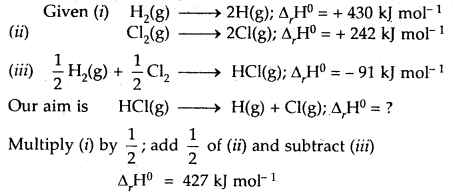Question 15.
CaIculat the enthalpy of hydrogenation of C2H2(g) to C2H4(g). Given bond energies: C—H = 414.50 kJ mol-1; C≡C is 827.6 kJ mol-1, C=C is 606.0 kJ mol-1; H—H = 430.5 kJ mol-1.= [827.6 + 2 × 414.0 + 430.5] – [606.0 + 4 × 414.0]
= 175.9 kJ mol-1.

Question 16.
The entropy change for the vaporization of water is 109 JK-1 mol-1. Calculate the enthalpy change for the vaporization of water at 373 K.
Δvap H = TΔvap S
= 373 × 109 = 40657 Jmol-1
= 40.657 kJ mol-1

Question 17.
Enthalpy and entropy changes of a reaction are 40.63 kJ mol-1 and 108.8 JK-1 mol-1 respectively. Predict the feasibility of the reaction at 27°C.
ΔH = 40.63 kJ mol-1 = 40630 Jmol-1
ΔS = 108.8 JK-1 mol-1
T = 27°C =27 + 273 = 300 K
Now ΔG = ΔH – TΔS
= 40630 – 3 × 108.8 = 7990 J mol-1

Since AG comes out to be positive (i.e., ΔG > 0), the reaction is not feasible at 27°C in the forward direction.

Question 18.
At 0°C ice and water are in equilibrium and ×H = 6.0 kJ mol-1 for the process H2O(s) → H2O(l). What will be ΔS and
ΔG for the conversion of ice to liquid water.?
Since the given process is in equilibrium
ΔG = 0
∴ from the equation ΔG = ΔH – TΔS
ΔH becomes = TΔS

∴ ΔS = $$\frac{6000 \mathrm{~J} \mathrm{~mol}^{-1}}{273 \mathrm{~K}}$$ = 21.98 JK-1 mol-1.

Question 19.
Calculate the standard- free energy for the reaction
4NH3(g) + 5O2(g) → 4NO(g) + 6H2O(l)
Given that the standard free energies of formation (ΔfG°) for NH3(g), NO(g), H2O(l) are – 16.8, + 86.7, and – 237.2 kJ mol-1 respectively. Predict the feasibility of the above reaction at the standard state.
Given ΔfG°(NH3) = – 16.8 kJ mol-1, ΔfG°(NO) = + 86.7 kj mol-1; ΔfG°(H2O) = – 237.2 kJ mol-1= – [4 × ΔfG°(NH3) + 5 × ΔfG°(O2)]
= [4 × 86.7 + 6(- 237.2)] – [4 × (- 16.8) + 5 × 0]
= – 1009.2 kJ
Since ΔrG° is negative, the reaction is feasible.

Question 20.
The value of K for the water gas reaction
CO + H2O ⇌ CO2 + H2 is 1.06 × 105 at 25°C. Calculate the standard free energy change for the reaction at 25°C.
ΔrG° = – 2.303 RT log K
= – 2.303 × 8.314 × 298 × log (1.06 × 105)
On solving, ΔrG° = – 28.38 KJ mol-1.

Question 21.
Calculate ΔrG° for the conversion of oxygen to ozone. $$\frac{3}{2}$$O2(g) → O3(g) at 298 K. If Kp for the reaction is 2.47 × 10-29
ΔrG° = – 2.303 RT log K
R = 8.314 JK-1 mol-1
∴ ΔfG° = – 2.303 (8-314 JK-1 mol-1) × 298 × (log 2.47 × 10-29)

On solving
ΔrG° = 163000 J mol-1 = 163 kJ mol-1

Question 22.
Find out the value of the equilibrium constant for the following reaction at 298 K. Standard Gibbs energy change, ΔrG° at the given temperature is – 13.6 kJ mol-1.
rG° = 2.303 RT log KQuestion 23.
For oxidation of iron, 4Fe(s) + 3O2(g) → 2Fe2O3 (s) entropy change is – 549.4 JK-1 mol-1 at 298 K. Inspite .of negative entropy change of this reaction, why is the reaction spontaneous? (ΔrH° = -1648 × 103 J mol-1).
The spontaneity of a reaction is determined from ΔStotal
(which is = Δsys + Δsutu)

Now ΔSsurr = – $$\frac{\Delta_{r} \mathrm{H}^{0}}{\mathrm{~T}}$$ at constant pressure
= $$\frac{-1648 \times 10^{3}}{298 \mathrm{~K}}$$ J mol-1 = 5530 JK-1 mol-1

Thus total entropy change for the reaction is
Δr Stotal = 5530 + (- 549.4) = 4980.6 JK-1 mol-1.
Since the total entropy change is positive, therefore, the above reaction is spontaneous.

Question 24.
A swimmer coming from a pool is covered with a film of water weighing about 18 g. How much heat must be supplied to evaporate this water at 298 K? Calculate the internal energy of vaporization at 100°C. AvapH° for water.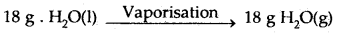No. of moles in 18 g H2O(l) = $$\frac{18 \mathrm{~g}}{18 \mathrm{~g} \mathrm{~mol}^{-1}}$$ = 1 mol.
Δvap U = Δvap H° – pΔV = ΔvapH – Δng RT (assuming steam behaving as an ideal gas)
Δvap H° – Δng RT = 40.66 kJ mol-1 – (1)(8.314 JK-1 mol-1)(373 K) (10-3 kJ) ,
∴ Δvap U° = 40.66 kJ mol-1 – 3.10 kJ mol-1 = 37.56 kJ mol-1

Question 25.
1 g or graphite is burnt in a bomb calorimeter in excess of oxygen at 298 K and 1 atm pressure according to the reaction
C(graphite) + O2(g) → CO2(g). During the reaction, the temperature rises from 298 to 299 K. If the heat, the capacity of the bomb calorimeter is 20.7 kJ K-1 what is the enthalpy change for the above reaction at 298 K and 1. atm?
= – $$\frac{12.0 \times(-20.7)}{1}$$ = – 2.48 × 102 kJ mol-1